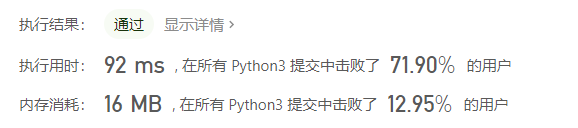## Leetcode每日一题：1208. 尽可能使字符串相等

2021/2/7 9:55:37 文章标签: 测试文章如有侵权请发送至邮箱809451989@qq.com投诉后文章立即删除

• 问题描述
• 思路分析及代码实现

## 问题描述

1 <= s.length, t.length <= 10^5
0 <= maxCost <= 10^6
s 和 t 都只含小写英文字母。

## 思路分析及代码实现

``````class Solution:
def equalSubstring(self, s: str, t: str, maxCost: int) -> int:
left = 0
right = 0
total = 0
res = 0
cha = []
for i in range(len(s)):
cha.append(abs(ord(s[i])-ord(t[i])))
while right < len(s):
total += cha[right]
if total > maxCost:
total -= cha[left]
left += 1
res = max(res, right-left+1)
right += 1
return res
``````暂无相关的数据...